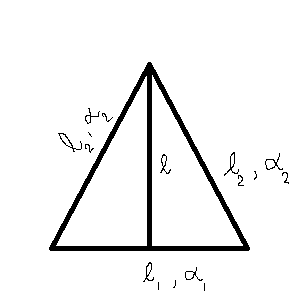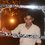# Why are the answers not same?

Here is a question,

An isosceles triangle is formed with thin rod of length ${ l }_{ 1 }$ and coefficient of linear expansion ${ \alpha }_{ 1 }$ as the base and two thin rods each of length ${ l }_{ 2 }$ and coefficient of linear expansion ${ \alpha }_{ 2 }$ as the two sides. If the distance between the apex and the midpoint of the base remain unchanged as the temperature is varied, find a relation between ${ l }_{ 1 }$, ${ l }_{ 2 }$, ${ \alpha }_{ 1 }$, ${ \alpha }_{ 2 }$.

Here is how I figured a relation,Case I

${ l }^{ 2 }=\quad { { \left( { l }_{ 2 } \right) } }^{ 2 }-{ { \left( \frac { { l }_{ 1 } }{ 2 } \right) } }^{ 2 }$

$\Rightarrow { \Delta l }^{ 2 }=\quad { { \left( { \Delta l }_{ 2 } \right) } }^{ 2 }-{ { \left( \frac { { \Delta l }_{ 1 } }{ 2 } \right) } }^{ 2 }$ -----$(1)$

${ \Delta l }_{ 2 }={ l }_{ 2 }-{ l }_{ 2 }\left( 1+{ \alpha }_{ 2 }\Delta T \right) ={ l }_{ 2 }{ \alpha }_{ 2 }\Delta T$ -----$(2)$

${ \Delta l }_{ 1 }={ l }_{ 1 }-{ l }_{ 1 }\left( 1+{ \alpha }_{ 1 }\Delta T \right) ={ l }_{ 1 }{ \alpha }_{ 1 }\Delta T$ ----$(3)$

$(2)$ & $(3)$ in $(1)$ and Since $\Delta l =0$,

${ \left( { l }_{ 2 }{ \alpha }_{ 2 }\Delta T \right) }^{ 2 }={ \left( \frac { { l }_{ 1 }{ \alpha }_{ 1 }\Delta T }{ 2 } \right) }^{ 2 }$

$\Rightarrow { l }_{ 2 }{ \alpha }_{ 2 }\Delta T=\frac { { l }_{ 1 }{ \alpha }_{ 1 }\Delta T }{ 2 }$

$\boxed{\Rightarrow \frac { { l }_{ 1 } }{ { l }_{ 2 } } =2\frac { { \alpha }_{ 2 } }{ { \alpha }_{ 1 } }}$

Case II

$\left( l \right) ^{ 2 }=\quad { { \left( { l }_{ 2 } \right) } }^{ 2 }-{ { \left( \frac { { l }_{ 1 } }{ 2 } \right) } }^{ 2 }$

Differentiating with respect to T,

$0=2{ l }_{ 2 }\frac { d{ l }_{ 2 } }{ dT } -\frac { 1 }{ 4 } 2{ l }_{ 1 }\frac { d{ l }_{ 1 } }{ dT }$

We know $d{ l }_{ 2 }={ { l }_{ 2 }\alpha }_{ 2 }\Delta T$ and $d{ l }_{ 1 }={ { l }_{ 1 }\alpha }_{ 1 }\Delta T$

$\Rightarrow { l }_{ 1 }.\frac { { { l }_{ 1 }\alpha }_{ 1 } }{ 2 } .\Delta T=2{ { { l }_{ 2 }l }_{ 2 }\alpha }_{ 2 }\Delta T$

$\boxed{\Rightarrow \frac { { l }_{ 1 } }{ { l }_{ 2 } } =2\sqrt { \frac { { \alpha }_{ 2 } }{ { \alpha }_{ 1 } } } }$Note by Anandhu Raj
5 years, 4 months ago

This discussion board is a place to discuss our Daily Challenges and the math and science related to those challenges. Explanations are more than just a solution — they should explain the steps and thinking strategies that you used to obtain the solution. Comments should further the discussion of math and science.

When posting on Brilliant:

• Use the emojis to react to an explanation, whether you're congratulating a job well done , or just really confused .
• Ask specific questions about the challenge or the steps in somebody's explanation. Well-posed questions can add a lot to the discussion, but posting "I don't understand!" doesn't help anyone.
• Try to contribute something new to the discussion, whether it is an extension, generalization or other idea related to the challenge.

MarkdownAppears as
*italics* or _italics_ italics
**bold** or __bold__ bold
- bulleted- list
• bulleted
• list
1. numbered2. list
1. numbered
2. list
Note: you must add a full line of space before and after lists for them to show up correctly
paragraph 1paragraph 2

paragraph 1

paragraph 2

[example link](https://brilliant.org)example link
> This is a quote
This is a quote
    # I indented these lines
# 4 spaces, and now they show
# up as a code block.

print "hello world"
# I indented these lines
# 4 spaces, and now they show
# up as a code block.

print "hello world"
MathAppears as
Remember to wrap math in $$ ... $$ or $ ... $ to ensure proper formatting.
2 \times 3 $2 \times 3$
2^{34} $2^{34}$
a_{i-1} $a_{i-1}$
\frac{2}{3} $\frac{2}{3}$
\sqrt{2} $\sqrt{2}$
\sum_{i=1}^3 $\sum_{i=1}^3$
\sin \theta $\sin \theta$
\boxed{123} $\boxed{123}$

Sort by:

@Anandhu Raj The relation you wrote as equation $1$ is incorrect. See, when you move from $l^{2} = {{ l }_{ 2 }}^{2} - \frac {{{ l }_{ 1 }}^{2}} { 4 }$ to the next step, you cant directly compare their changes the same way.

Since $l$ always remains constant, therefore, after sometime, the new relation will be:

${ l }^{ 2 }={ ({ l }_{ 2 }+\Delta { l }_{ 2 }) }^{ 2 }-\frac { ({ { l }_{ 1 }+\Delta { l }_{ 1 } })^{ 2 } }{ 4 }$

Now, equating these two lengths, you get:

${ { l }_{ 2 } }^{ 2 }-\frac { { { l }_{ 1 } }^{ 2 } }{ 4 } ={ ({ l }_{ 2 }+\Delta { l }_{ 2 }) }^{ 2 }-\frac { ({ { l }_{ 1 }+\Delta { l }_{ 1 } })^{ 2 } }{ 4 } \\ { l }^{ 2 }-\frac { { { l }_{ 1 } }^{ 2 } }{ 4 } ={ { l }_{ 2 } }^{ 2 }+{ \Delta { l }_{ 2 } }^{ 2 }+2{ l }_{ 2 }\Delta { l }_{ 2 }-\frac { { l }^{ 1 }+{ \Delta { l }_{ 1 } }^{ 2 }+2{ l }_{ 1 }{ \Delta l }_{ 1 } }{ 4 }$

Now, since, we consider $\Delta { l }_{ 1 }$ and $\Delta { l }_{ 2 }$ to be negligible, their squares are neglected. Hence, we are left with the relation:

$4{ l }_{ 2 }\Delta { l }_{ 2 }={ l }_{ 1 }\Delta { l }_{ 1 }$

Now, using the relation, $\Delta { l }_{ 1 }={ l }_{ 1 }{ \alpha }_{ 1 }\Delta T$ and $\Delta { l }_{ 2 }={ l }_{ 2 }{ \alpha }_{ 2 }\Delta T$, we get:

$4{ l }^{ 2 }{ { \alpha }_{ 2 } }={ { l }_{ 1 } }^{ 2 }{ \alpha }_{ 1 }\\ \frac { { l }_{ 1 } }{ { l }_{ 2 } } =2\sqrt { \frac { { \alpha }_{ 2 } }{ { \alpha }_{ 1 } } }$

:)

- 5 years, 4 months ago

Thanks! :)

- 5 years, 4 months ago